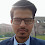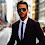## Sunday, May 17, 2015

### Primer: Par And Zero Coupon Yield Curves

Par and zero coupon curves are two common ways of specifying a yield curve. Par coupon yields are quite often encountered in economic analysis of bond yields, such as the Fed H.15 yield series. Zero coupon curves are a building block for interest rate pricers, but they are less commonly encountered away from such uses.

## The Zero Curve And Discount Curve

In order to avoid being dragged down with the details of fixed income pricing conventions, this article uses a basic interest rate convention, and only looks at maturities which are an integer number of years.

The chart above shows the example zero coupon curve that is used within this article. The top panel shows the zero coupon rate for maturities running from 0,1,...,10 years from the calculation date. The bottom panel shows the implied discount factors for each of those dates. Note that we can define yield curves for any issuer of bonds (for example, the U.S. Treasury), or for derivatives such as swaps. Within a single currency, there are often several yield curves of interest.

The relationship between the zero rate and the discount factor is:

DF(t) = 1/(1+r)^t,

where DF is the discount factor, and r is the zero rate for maturity t (in years). One of the important properties of the discount factor is that it is equal to 1 at t=0. (Older textbooks would also say quaint things such as the fact that the discount factor will be less than 1 for t>0, as negative rates are allegedly impossible.)

The interpretation of the discount factor is that it is the present value of receiving \$1 at a future date. or example, the zero rate at t=10 is 6%, and the associated discount factor is equal to 1/(1.06)^10 = 0.5584. This means that we would be willing to pay \$0.5584 now to receive \$1 in 10 years (and receive a rate of return of 6%.)

For real world pricing applications, the zero curve is continuous, and defined for every day from overnight maturities to some maximum maturity. The difficulty with defining the curve for every day is defining the time-to-maturity: how do we account for things like leap years, non-working days, etc.

Note that there are other conventions for quoting a zero rate. If you are developer who works with pricing software, it is a safe bet that different libraries use different conventions. Therefore, the only safe way of comparing the two yield curves is by using the discount factor curve, and not the zero rates.

It is possible to buy zero coupon bonds, which only pay a cash flow at maturity (these are known as strips).  The price of a zero coupon bond would correspond to the discount factor. However, this market is not particularly liquid, and so is of limited interest to institutional investors.

## Pricing A Coupon Bond And The Par Curve

We now look at bonds that pay a coupon (which are the standard instruments). For simplicity, I am looking at bonds that pay coupons annually. If the coupon rate is C, and has a maturity T, the bond cash flows are:
• pays \$C every year, up to and including the year T; and
• pays \$1 on year T (the principal repayment).
We can then calculate the present value of all off those cash flows by multiplying them by the appropriate discount factors. (An example is given below.)

The chart above shows the present value of 10-year bonds (using the example yield curve) as a function of the coupon rate. The higher the coupon, the more valuable the bond, We see that the bond has a price of \$100 when the coupon rate is 5.88% (roughly). Since we say that a bond that is trading with a price of \$100 is trading at par, we then say that 5.88% is the 10-year par coupon yield. That is, if the issuer associated with the yield curve issued a bond with a coupon of 5.88%, it would trade at par.

Time Zero Rate Discount Factor Cash Flow Present Value
1
5%
0.952
5.88
5.60
2
5%
0.907
5.88
5.33
3
5.125%
0.860
5.88
5.06
4
5.25%
0.815
5.88
4.79
5
5.375%
0.770
5.88
4.52
6
5.5%
0.725
5.88
4.26
7
5.625%
0.682
5.88
4.01
8
5.75%
0.639
5.88
3.76
9
5.875%
0.598
5.88
3.51
10
6%
0.558
105.88
59.12

The table above shows the calculations for the 10-year par coupon yield. Within each row, the cash flow is multiplied by the discount factor to get the Present Value of the cash flow. The sum of the present value of all of the cash flows is \$100 (within rounding errors).

The chart above shows the par coupon yields, as well as the zero rates for maturities from 0 to 10.

For the first 2 years, the zero rate and the par coupon yield are the same, as the curve was flat at 5%. (This is the result of my choice of calculation conventions; if we look at bonds that pay semi-annually, the par coupon yield differs from the zero rate, even if the curves are flat.)

For longer maturities, the par coupon yield is below the corresponding zero rate. These bonds have coupons that are paid before maturity, and they are discounted at a lower rate than the zero rate at the maturity of the bond. This is what you expect to see with a positive yield curve slope (rates rising for longer maturities). Buying zero coupon bonds to maximise the yield of your portfolio is a common tactic.

## Where Do These Curves Come From?

In order to generate the zero curve, we typically assume that is a function of a particular form, and we then fit the function parameters to the prices of traded instruments. In the case of bonds, what we typically find is that bonds will not sit perfectly upon such a curve. The end result is that if we compare the traded prices of bonds relative to what is predicted by discounting using our fitted zero curve, some bonds will be expensive, and others cheap.

## Par Coupon Versus Benchmark Yields

An popular alternative for quoting generic bond yields is to use a benchmark bond yield. A benchmark bond is the most heavily traded instrument at a particular maturity; for the U.S. Treasury, it is the latest security issued at a particular maturity. In other markets, the determination of which issue is the benchmark is slightly more complex. (New issues start out with a small amount outstanding, and then the outstanding  amount is increased until it gains benchmark status.)

Since the benchmarks are heavily traded, they are followed widely. This can cause confusion, as benchmark bonds are often expensive relative to the curve, and so have a lower yield than the corresponding par coupon yield. For example, during the LTCM crisis, a pretty wide spread opened up between the benchmark 10-year Treasury Note yield and the 10-year par coupon yield.

Although it may be sensible to make use of the benchmark yield in a pricing application, it is not a good idea for time series analysis. New benchmark issues typically start trading at a different yield than the old benchmark, and so there is a jump every time the benchmark rolls. This creates discontinuities in benchmark yield series that do not correspond to market movements. Par coupon curves are not greatly affected by the appearance of new benchmarks, and so movements in the par coupon time series are a closer match to true market movements.

(c) Brian Romanchuk 2015

1.2.This is missing the point as the term "par rate" is never explained but plotted on a graph ... ???

1.Well, now that you raised the point, I should have broken the definition out more. Howver, it is within the text. The par rate is the yield on a bond that is trading at par (and is fairly valued on the discount curve).

2.Par curve is a curve which tells us for a given maturity what the coupon rate should be to make the bond priced at par (At par coupon = YTM). Par rate is used for discounting all the CFs of a bond (not just one single). 5Y par curve is the rate that you will use to discount all the cash flow of the given 5Y bond.

3.This comment has been removed by a blog administrator.

4."Note that there are other conventions for quoting a zero rate. If you are developer who works with pricing software, it is a safe bet that different libraries use different conventions. Therefore, the only safe way of comparing the two yield curves is by using the discount factor curve, and not the zero rates."

1.I don't have references handy, but the logic is as follows. Take the 1 year tenor. The discount factor could be 0.99 (\$1 in 1 year = \$0.99 now). That can be mapped to a number of different discount rates.
- 0.99 = 1/(1+r)^1.
- 0.99 = e^{-r1}.
- Whatever the market quote convention is for 1-year zeros, taking into account day count conventions, semi-annual/annual conventions for coupon bonds, and so forth.

The last possibility might be fairly common, as pricing software might want the quoted curve to align to what market makers see.

However, in all cases, the price is the same 0.99.

5.This comment has been removed by a blog administrator.

6.This comment has been removed by a blog administrator.

7.This comment has been removed by a blog administrator.

8.Hi, why is it the first year par rate is equal to zero rate?

1.The zero curve starts out flat at 5%. For the the quote convention I used for annual pay bonds, the zero rate is the same as the par coupon rate. If we look at semi-annual bonds, this will not be true, since there is a coupon at six months, and that affects the compounding,

It is rather easy to see that a one year zero should be the same as a one year annual par coupon. Both have a single payment one year from now. The only difference is that the zero is just principal, while the par coupon is principal plus coupon. This just creates a cosmetic difference, but since you are paying at t=0 for a single cash flow at t=1, the economic return should be the same. (This is why semi-annual bonds are different; they have an intermediate cash flow at six months as well.)

9.This comment has been removed by a blog administrator.

10.This comment has been removed by a blog administrator.

11.This comment has been removed by a blog administrator.

12.This comment has been removed by a blog administrator.

13.This comment has been removed by a blog administrator.

14.This comment has been removed by a blog administrator.

Note: Posts are manually moderated, with a varying delay. Some disappear.

The comment section here is largely dead. My Substack or Twitter are better places to have a conversation.

Given that this is largely a backup way to reach me, I am going to reject posts that annoy me. Please post lengthy essays elsewhere.